# Learning The Basics Of Series and Parallel resistance [Quick Guide]Hi, hope you are having a good life. In this post, I am going to share with you my knowledge about series and parallel resistance. I will share the very basic points which I wish someone taught me when I was learning the basics of electronics.

In designing an electronic circuit, a resistor is probably the most important and commonly occurring component. Almost every portable circuits contain very complex combinations of resistors.

Therefore, it is very useful to have a set of rules to find out the equivalent resistances for some common and general arrangements of resistor elements.  And it is found that such simple rules are parallel series combinations.

First of all, let me clear a basic confusion. Series parallel combinations are just like series and parallel circuits but they are made of only resistor elements. In fact, most of the points you will learn here will also apply to any series and parallel circuit.

In summary, series and parallel circuits are general terms, and series-parallel resistance is specific circuits with only resistors.

## Series Resistance

Resistors, no matter of what sizes, connected in such a way that the same current flows through each of them. The equivalent resistance of such connected resistors is known as series resistance.

In series connected resistance/resistors there is only one path for electric current to flow. All resistors are connected in a row like links of a chain. When one of the links of the chain breaks the whole chain becomes useless. Same is the case with series resistors when one resistor burns out the whole circuit stops to operate.

Current remains same at any node in series resistance circuit. You can prove it practically by using a multimeter and the above circuit made on a breadboard. Or by just simulating the circuit (Figure: 1) in any simulation software, as I did it in NI Multisim.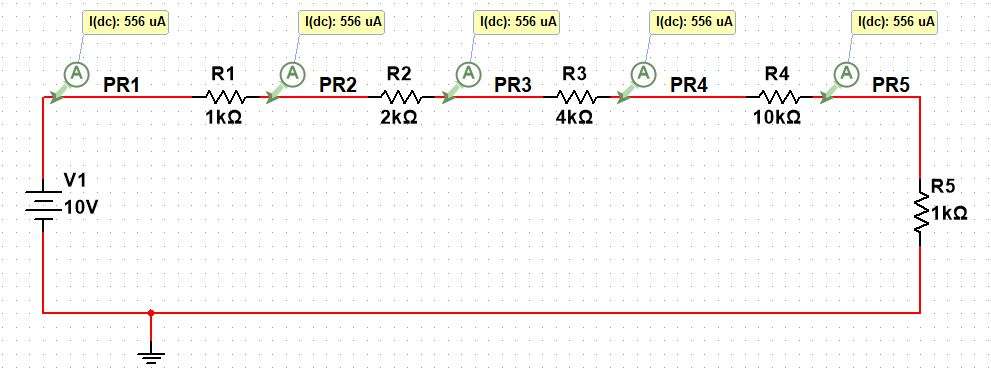### How to find total series resistance?

All resistors connected in series are added to give equivalent series resistance. The general formula is given below:$R_{{eq}}=R_{{1}}+R_{{2}}+R_{{3}}+R_{{4}}+ . . . . . + R_{{N}}$

To find the equivalent series resistance, remove any voltage source from the circuit, and then sum up the values of each resistor present in the circuit. Let take the example of the above circuit (Figure: 1).$R_{{eq}}=R1+R2+R3+R4+R5 \\ R_{{eq}}= 1k\Omega +2k\Omega +4k\Omega +10k\Omega +1k\Omega \\ R_{{eq}}= 18k\Omega$

Series resistance may be connected to a current source. Same steps go for the current source as well. Remove the current source and sum up the resistors values. An example is given below: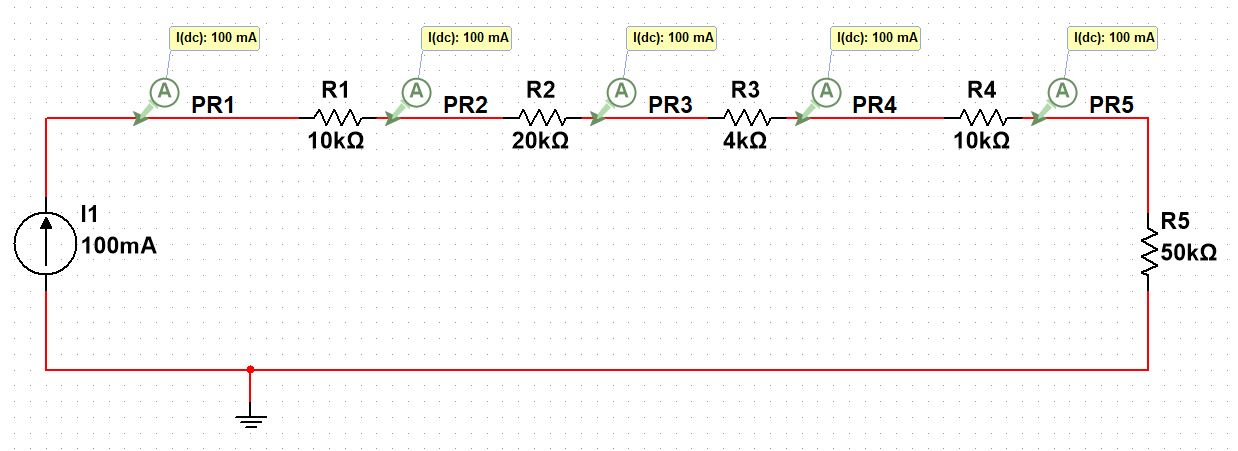Mathematical calculation:$R_{{eq}}=R1+R2+R3+R4+R5 \\ R_{{eq}}= 10k\Omega +20k\Omega +4k\Omega +10k\Omega +50k\Omega \\ R_{{eq}}= 94k\Omega$

A circuit may take any shape. But Resistors must be connected just like links of chain. Following circuit is a series connected.

Can you prove it?

Can you find it equivalent series resistance? (Answer: 378KOhm)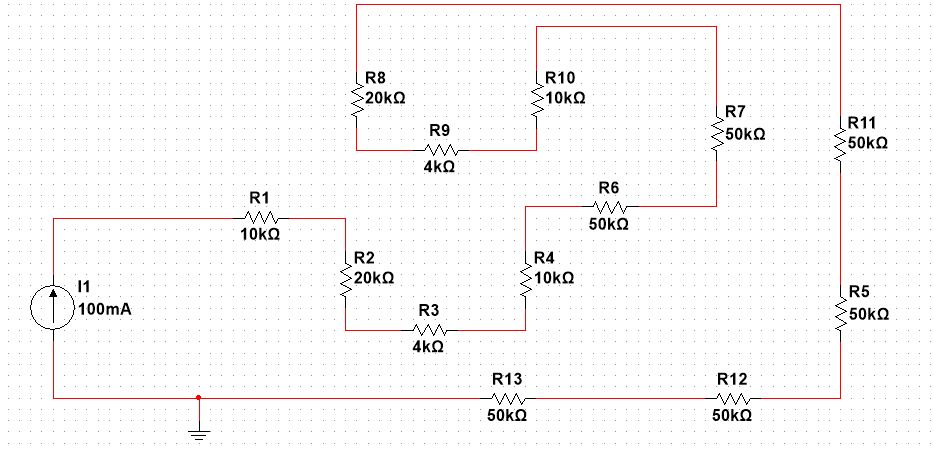A good point to remember is, series connected resistors share only one common node with each other. It is the very important point to understand. Later on in this post, I will share an example circuit with series and parallel combinations combine.

It will be confusing a little bit then. So pay close attention now because this point will help us to identify a series combination of resistors in a complex circuit.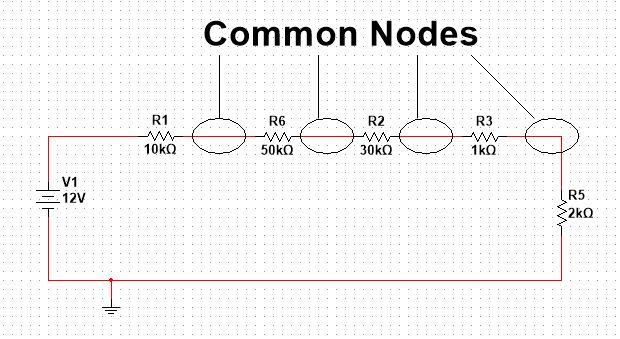## Parallel Resistance

Resistors, no matter of what sizes, connected in such a way that each resistor have the same voltage across it.  The equivalent resistance of such connected resistors is known as parallel resistance.

Or parallel resistance can be defined as resistors are in parallel if the nodes at both ends of the resistors are the same. In parallel connected resistors (Parallel resistance) there are different paths for electric current to flow. The amount of current flow depends on the values of resistors.

In parallel, the equivalent resistance is always less than the smallest individual resistor. For example, the equivalent resistance of the above circuit will always be less than 1KOhm. And the voltage across each resistor is 12V as all resistor are connected to the same nodes as that of 12V.

I was talking about resistors are in parallel if the nodes at both ends of the resistors are the same.  let me show the common nodes in the above circuit.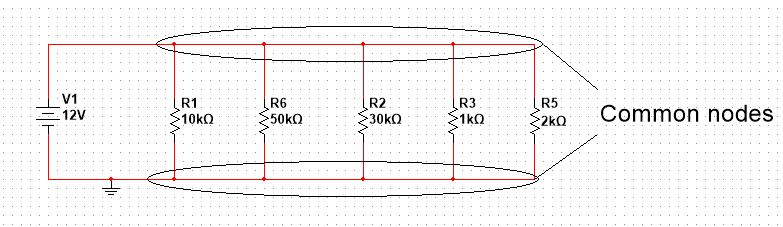### How to find total parallel resistance?

In parallel connected resistors, equivalent resistance is equal to the addition of reciprocal values of each resistor. The general formula for equivalent parallel resistance is given below:$\frac{1}{R_{eq}}=\frac{1}{R_{1}}+\frac{1}{R_{2}}+\frac{1}{R_{3}}+\frac{1}{R_{4}}+....+\frac{1}{R_{N}}$  Let find the value of total resistance for the above circuit.

Remove the voltage source and then put the values of resistors in the above general formula.$\frac{1}{R_{eq}}=\frac{1}{10k\Omega }+\frac{1}{50k\Omega }+\frac{1}{30k\Omega }+\frac{1}{1k\Omega }+\frac{1}{2k\Omega }\\ \frac{1}{R_{eq}}=1.6533m\Omega\\ R_{eq}=604.85\Omega$

The answer is less than the smallest individual resistor.

Let’s have another example: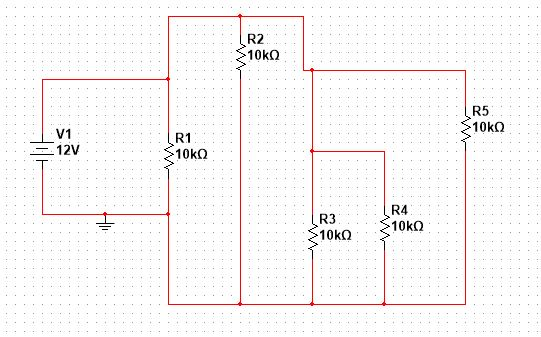Can you tell me how is this circuit even a parallel?

Of course this is a parallel connected circuit.

It is following the criteria of, resistors are in parallel if the nodes at both ends of the resistors are the same.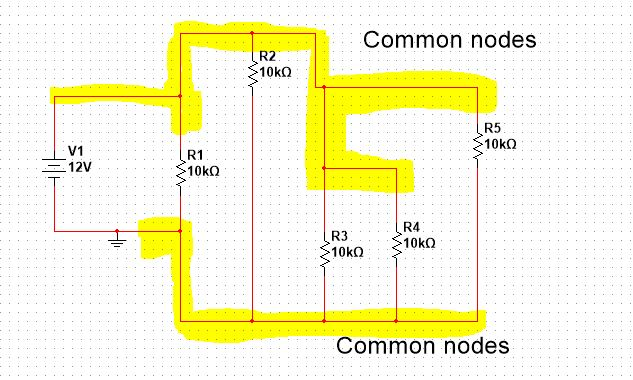The shape confused us, right? But still, it is a parallel connected resistive circuit. The point I am saying. It is not important what shape circuit may have, you must know the basic concept of how to identify the parallel resistors in a complex circuit.

We can redraw the above circuit in a clean way as below: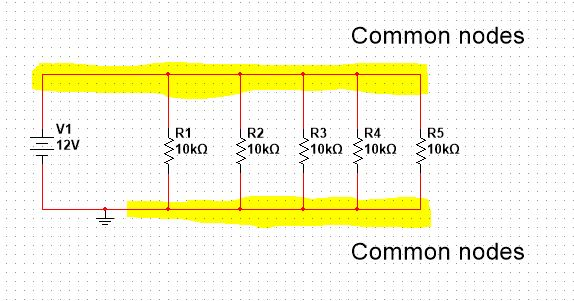### A quick trick to find the equivalent parallel resistance

When all parallel connected resistors have the same values, equivalent parallel resistance can be found by just dividing the same value by the total number of resistors. The general formula can be written like this:$R_{eq}=\frac{Common\,value}{Total\,\,number\,\, of\,\, resistors}$

Let apply this quick trick on the above circuit.$R_{eq}=\frac{Common\,value=10k\Omega}{Total\,\,number\,\, of\,\, resistors=5}\\ R_{eq}=\frac{10k\Omega}{5}\\ R_{eq}=2k\Omega$

It would be good if you take some time to prove it by using the general formula for parallel resistance. It will be interesting to see both methods will have the same answer.

## Differences between series and parallel resistance

I do not know how much differences are there between the said resistances in total. Following are the differences which I learned with time.

1. In series resistance same current flows through each resistor while in parallel resistance each resistor has the same voltage across it.

2. In series resistance, total voltage is equal to a voltage drop across each resistor. See in the following diagram: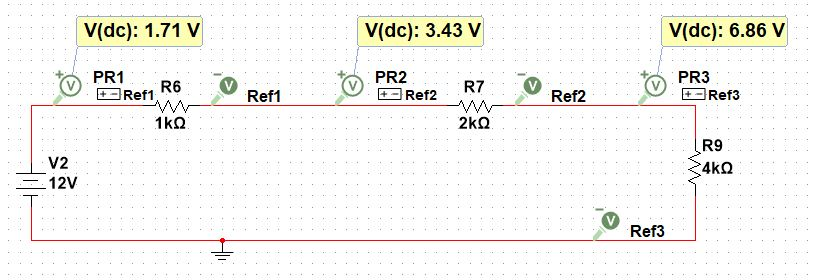The total voltage applied is 12V (battery source), which means the total sum of voltages across each resistor must be equal to 12V.$V_{total}=V_{1k\Omega}+V_{2k\Omega}+V_{4k\Omega}\\ V_{total}=1.71V+3.43V+6.86V\\ V_{total}=12V$

Let’s try to solve the above circuit manually.

Resistors are connected in series. The total series equivalent resistance can be found by using general formula for series resistance.$R_{eq}=1k\Omega+2k\Omega+4k\Omega\\ R_{eq}=7k\Omega$

Using Ohm’s law, total current can find out as follows:$V_{total}=IR_{eq}\\ I=\frac{V_{total}}{R_{eq}}\\ I=\frac{12V}{7k\Omega}\\ I=1.72mA$

We calculated total current. To find the voltage drop across each resistor, multiply the total current with resistor value (Ohm’s Law).$V_{1k\Omega}=IR_{1k\Omega}=(1.72mA)(1k\Omega)=1.72V\\ V_{1k\Omega}=IR_{2k\Omega}=(1.72mA)(2k\Omega)=3.40V\\ V_{1k\Omega}=IR_{4k\Omega}=(1.72mA)(4k\Omega)=6.88V$

We got the exact same values as were given by Multisim simulation. Adding all the calculated voltages will end in 12V. You can prove it by adding them yourself using a calculator.

3. In parallel resistance, the total current is equal to a sum of currents flowing through each resistor.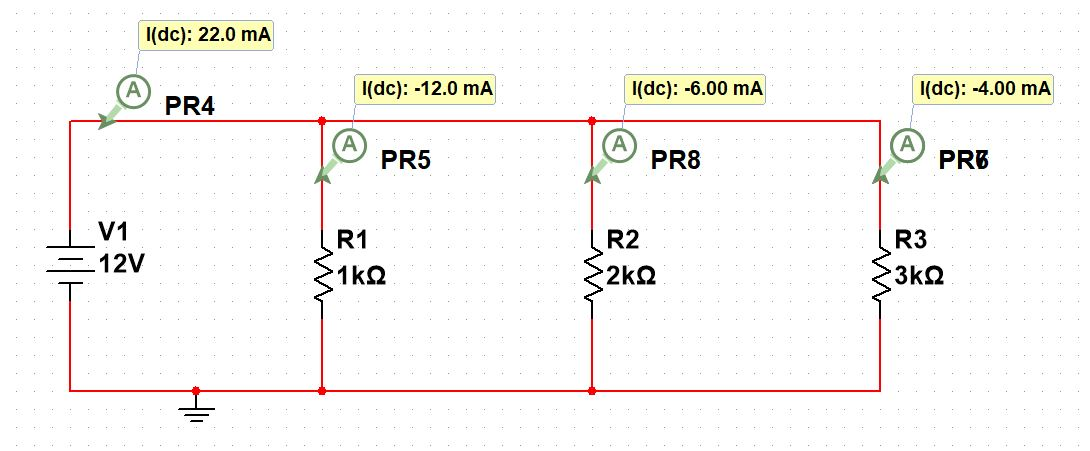Total current (22.0mA) is equal to a sum of the current flowing from each resistor. You can prove it by adding them together using a calculator. Do not confuse with the negative sign. Ignore it.

4. In series resistance, if one resistor fails to operate properly, rest of the circuit also fails to operate. In parallel, the failing of one resistor does not affect the whole circuit.

5. Voltage drop in series resistance is proportional to the size of the resistor. While in a parallel the amount of current flow in each resistor depends on the size of a resistor. Increasing the size of the resistor increases voltage drop as well as current flow.

## Why do we actually need these combinations?

There are very important applications of series and parallel combinations. Right now I am sharing what I know and will update it as I learn more about it.

I was working on a circuit and I needed a 2kOhm resistor which I did not have at the time. I searched a lot in my small lab but all in vain. Then I put together two 1kOhm resistors to make a 2kOhm resistor. I did it by placing two 1kOhm resistors in series.

I think you get the point. Series and parallel can be very helpful when you do not have the exact value resistor with you. It may also be helpful if you are designing a circuit and you need a resistor value which is not available in near market. You can make the desired value of the resistor by playing with resistor series and parallel combinations.

These combinations also find applications in the design of Voltmeter and Ammeter respectively.

Series resistance is used as a current limiter in circuits. It can be used as a voltage divider for an LED circuit. You may have seen every led has a resistor in series. The job of the resistor is to limit the current flowing through the LED.

## Example

Let’s have an example to clarify our concept of parallel series combinations even more. The circuit in the example has a mixed parallel and series combinations. So please pay close attention and try to solve at each step.

My recommendation is, bring the notebook, calculator and a good pen. And let try to solve with me.## Summary

• Resistors connected in such a way that same current flows through each resistor, then the equivalent resistance of the resistors is called series resistance.
• In series, current remain same no matter what the sizes of resistors. And this is only possible when resistors are connected like links of a chain.
• In parallel connected resistors, the voltage remains same across each resistor no matter what is the value of each resistor.

I hope this post was helpful to you. This is what I know about the series and parallel resistance. And I will keep updating this post as I learn something new about the mentioned topic.

Thank you so much for reading and have a good life.

Other useful posts

Scroll to Top